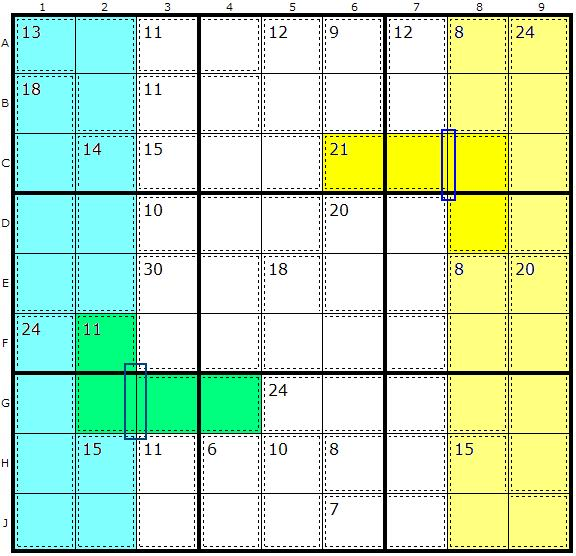Main Page - Back

# Cage Splitting

From sudokuwiki.org, the puzzle solver's siteWith Innies and Outies, we were looking out for single cells poking into a block or protruding outwards. We said that a block can be any combination of adjacent units (as long as they don’t overlap, like a row and a column). Sometimes, there just won’t be a single cell in one cage.In this example, which is the "Tough" example on the Killer Solver, we have two cage splitting examples. A side note: because the cage pattern is symmetrical you should be able to find and use this strategy twice. Not all Killer puzzles are symmetrical, however, but it is worth looking out for.

Lets look at the yellow block - a 2 column block. We can see the straight line dividing columns 7 and 8 and just one place where a cage pokes out. The sum of all the yellow cages - less the outie - is 75. So we now know that C8 and D8 must add up to 15 (90-75). This allows us to split the cage into two 2-cage cells, 15 and 21-15=6, the outie half. So 3,6,7,8 and 9 can be removed from C6 and C7 and 1,2,3,4 and 5 can be removed from C8 and D8.

Similarly, the 2-column blue block has a symmetrically placed outie 4-cage summing to 11. The split gives us 6 as the value of the innie (F2,G2) and 5 as the value of the outie (G3,G4).

Cage Splitting doesn’t often get us whole solutions, but together with combinations (our first strategy), it can dramatically reduce the possibilities in cells. Cage Splitting can leave behind an innie or an outie elsewhere in another direction, which is also useful.

## Using Locked Sets to Split Cages

In trying to understand an idea kindly emailed to me I got a different brain wave altogether looking at the same puzzle. Cage Splitting, as described above, works by finding one cage that is half in and half out of a block. This limits the chances of finding an instance because either quite a few cages need to be lined up or many cells need to be solved. What if there were a way to expand the number of Cage Splits?